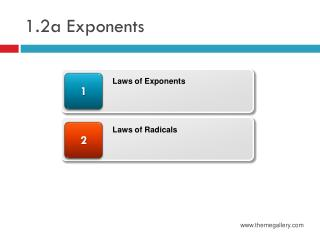DownloadDownload Presentation1.2a Exponents

# 1.2a Exponents

Download Presentation## 1.2a Exponents

- - - - - - - - - - - - - - - - - - - - - - - - - - - E N D - - - - - - - - - - - - - - - - - - - - - - - - - - -
##### Presentation Transcript

1. 1.2a Exponents 1 Laws of Exponents 2 Laws of Radicals www.themegallery.com

2. Exponents • Notation • an = a*a*a*a….*a, n times • a4 = a*a*a*a • a9 = a*a*a*a*a*a*a*a*a • 34 = 3*3*3*3 = 81 • can = c*a*a*a…*a, n times • 13a3, where a =2 =13*2*2*2 =13*8 =104 www.themegallery.com

3. Exponents • Laws of Exponents • anam = an+m • 2329 = 23+9 = 212 = 4096 • Note: the base number (a) must be the same • (am)n = amn • (23)4 = 23*4 = 212 = 4096 • (ab)n = anbn • (a/b)n = an/bn • (2/5)3 = 23/53 = 8/125 www.themegallery.com

4. Exponents • Laws of Exponents (Cont.) • am/an = am-m • 25/23 = 25-3 = 22 = 4 • a-m = 1/am • x-3 = 1/x3 • a-m/b-n = bn/am • X-5/y-4 = y4/x5 www.themegallery.com

5. Radicals • Notation: • Note: • Properties • if is a real number • , • if a≥0 www.themegallery.com